# Which of the following is a correct way to typecast an integer to a double? double(x)...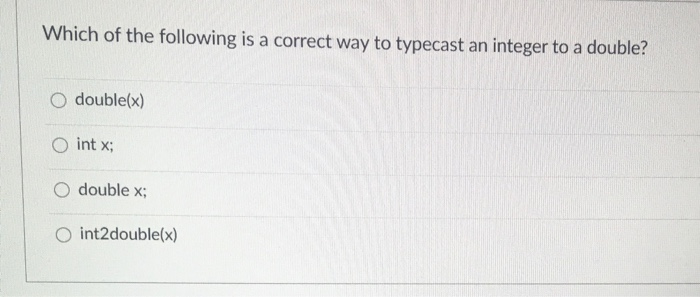Which of the following is a correct way to typecast an integer to a double? double(x) int x; O double x; int2double(x)

SOL:

so First option  is correct answer

Explanation:

Typecasting means we are converting one datatype to another datatype

we want to convert a integer to double so we keep integer variable in braces and before that double datatype is placed

so double(x) is the correct answer

#### Earn Coin

Coins can be redeemed for fabulous gifts.

Similar Homework Help Questions
• ### Which one is correct? Thanks. Please give a simple explanation. Assuming that class X has a...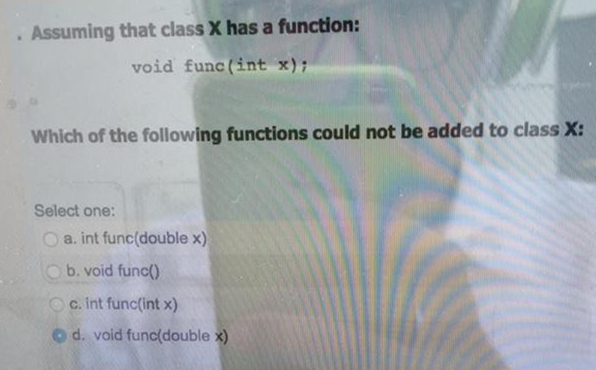Which one is correct? Thanks. Please give a simple explanation. Assuming that class X has a function: void func (int x) Which of the following functions could not be added to class x: Select one: a. int func (double x) b. void func() c. int func (int x) o d. void func(double

• ### (5.00 Points) 11 - The function prototype double mySqrt(int x); defines a function called a) double...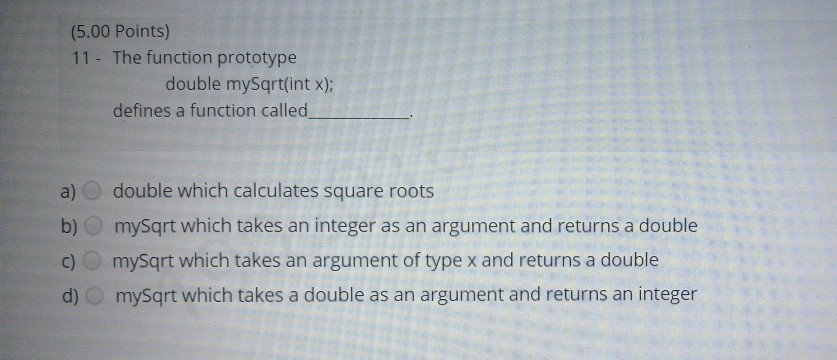(5.00 Points) 11 - The function prototype double mySqrt(int x); defines a function called a) double which calculates square roots b) mySqrt which takes an integer as an argument and returns a double c) mysqrt which takes an argument of type x and returns a double d) mysqrt which takes a double as an argument and returns an integer

• ### what are the answes Which yields 2.5? (double)(10/4) (int)(10) / (int)(4) 10/4 (double)(10) / (double)(4) Considering...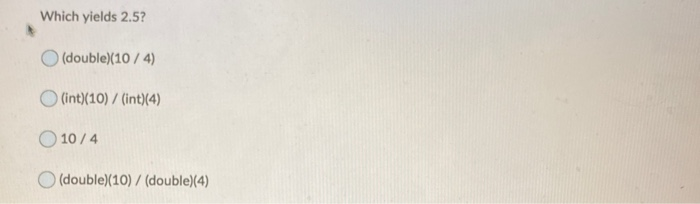what are the answes Which yields 2.5? (double)(10/4) (int)(10) / (int)(4) 10/4 (double)(10) / (double)(4) Considering integer division, determine the result of 13 / 3 4.3 05 4.0 4 Which of the following statements is a valid declaration of a constant integer variable named STEP_SIZE? int STEP_SIZE = 5; O final int STEP_SIZE = 14; double STEP_SIZE = 5; final STEP_SIZE = 10; Which is an invalid identifier? num_Cars1 3numCars O_numCars numCars Which one is not a programming language. Java...

• ### For two given array declarations: int x and double y, which of the following is FALSE?...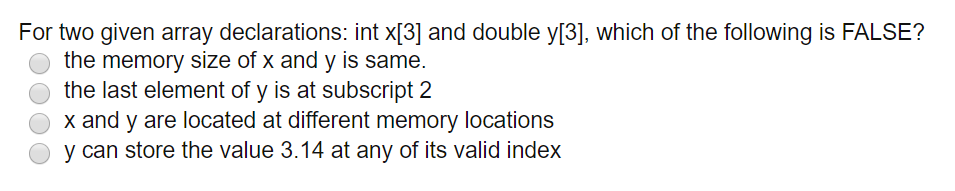For two given array declarations: int x and double y, which of the following is FALSE? o the memory size of x and y is same. O the last element of y is at subscript 2 O x and y are located at different memory locations o y can store the value 3.14 at any of its valid index

• ### Given an integer x which is assumed to be in the list a, write a method...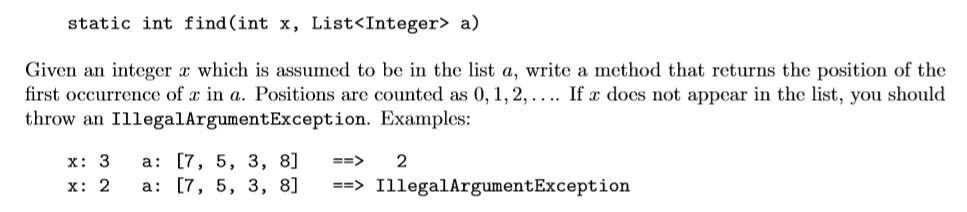Given an integer x which is assumed to be in the list a, write a method that returns the position of the ﬁrst occurrence of x in a. Positions are counted as 0,1,2,.... If x does not appear in the list, you should throw an IllegalArgumentException. static int find(int x, List<Integer> a) Given an integer x which is assumed to be in the list a, write a method that returns the position of the first occurrence of u in a....

• ### Show the symbol table and activation record for the following function. double cubic(double x, int a,...

Show the symbol table and activation record for the following function. double cubic(double x, int a, int b, int c, int d) {     double y = 0.0;     y = a*x*x*x + b*x*x + c*x + d;     return y; }

• ### Which of the following verifies that the user enters a positive integer value? A. int num...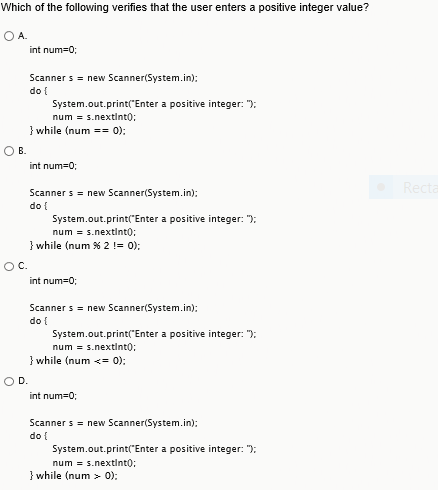Which of the following verifies that the user enters a positive integer value? A. int num = 0: Scanner s = new Scanner(System.in): do { System.out.print("Enter a positive integer: "): num = s.nextlnt(): } while (num = = 0): B. int num=0: Scanner s = new Scanner(System.in): do { System.out.print("Enter a positive integer: "): num = s.nextlnt(): } while (num % 2 ! = 0): C. int num=0: Scanner s = new Scanner(System.in): do { System.out.print("Enter a positive integer: "):...

• ### Consider the following program: # include <iostream> using namesapce std; void Func(int a, int bl double...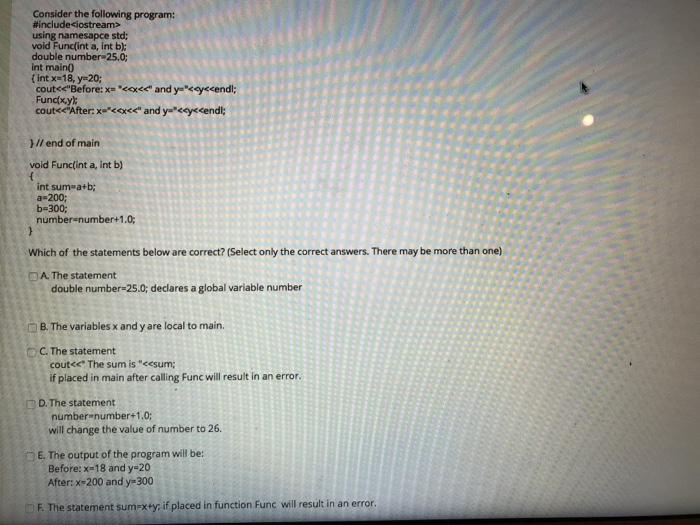Consider the following program: # include <iostream> using namesapce std; void Func(int a, int bl double number-25.0: int main) f int x-18, y-20; cout<c"Before: x- kex<" and y-eyecendl; Fundxy 1// end of main void Funcfint a, int b) int sum a+b; a-200; b-300; numberanumber+1.0 Which of the statements below are correct? (Select only the correct answers. There may be more than one) D A The statement double number-25.0; declares a global variable number B. The variables x and y are...

• ### For any positive integer n, prove that .Hint for one way of proving this: If X...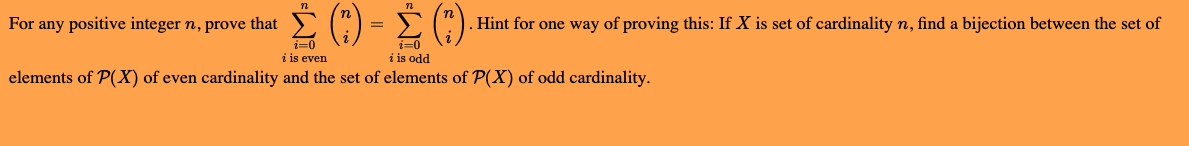For any positive integer n, prove that .Hint for one way of proving this: If X is set of cardinality n, find a bijection between the set of o i is even i is odd elements of P(X) of even cardinality and the set of elements of P(X) of odd cardinality.

• ### Fix each of the following code by converting the type of the variables: a. double x...

Fix each of the following code by converting the type of the variables: a. double x = 1.5; int y = x b. String x = "1"; Int y = x; c. int x = 1; String y = x; d. double x = 1.0; String y = x;